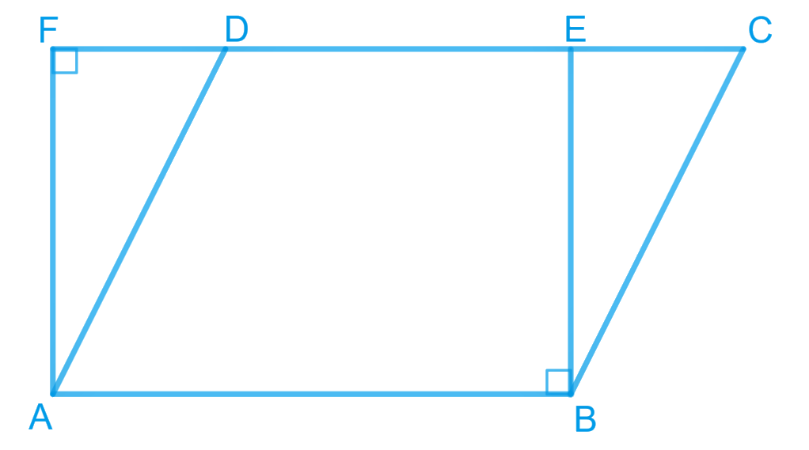# Ex.9.4 Q1 Areas of Parallelograms and Triangles Solution - NCERT Maths Class 9

Go back to  'Ex.9.4'

## Question

Parallelogram $$ABCD$$ and rectangle $$ABEF$$ are on the same base $$AB$$ and have equal areas. Show that the perimeter of the parallelogram is greater than that of the rectangle.

Video Solution
Areas Of Parallelograms And Triangles
Ex 9.4 | Question 1

## Text Solution

What is known?

Parallelogram $$ABCD$$ and rectangle $$ABEF$$ are on the same base $$AB$$ and have equal areas.

What is unknown?

How we can show that the perimeter of the parallelogram is greater than that of the rectangle.

Reasoning:

When we compare the sides of rectangle and parallelogram which are on same base, we can see two sides of parallelogram are opposite to $$90$$ degree which shows that these two are longer than rectangle two sides and as we find the perimeter of both quadrilateral, we will get the required result.

Steps:

As the parallelogram and the rectangle have the same base and equal area, therefore, these will also lie between the same parallels.

Consider the parallelogram $$ABCD$$ and rectangle $$ABEF$$ as follows.

##Here, it can be observed that parallelogram $$ABCD$$ and rectangle $$ABEF$$ are between thesame parallels $$AB$$ and $$CF$$.

We know that opposite sides of a parallelogram or a rectangle are of equal lengths. Therefore,

\begin{align}&{AB}={EF} \text { (For rectangle) } \\ &{AB}={CD}(\text { For parallelogram }) \\ &\therefore {CD}={EF} \\ &\therefore {AB}+{CD}={AB}+{EF} \quad \ldots(1)\end{align}

Of all the line segments that can be drawn to a given line from a point not lying on it, the perpendicular line segment is the shortest.

$\therefore {AF}<{AD}$

And similarly, $$BE < BC$$

$\therefore {AF}+{BE}<{AD}+{BC}\quad \ldots(2)$

From Equations ($$1$$) and ($$2$$), we obtain

\begin{align} &{AB}+{EF}+{AF}+{BE} \\ & <{AD}+{BC}+{AB}+{CD} \end{align}

Perimeter of rectangle $$ABEF$$ $$<$$ Perimeter of parallelogram $$ABCD$$.

Video Solution
Areas Of Parallelograms And Triangles
Ex 9.4 | Question 1

Learn from the best math teachers and top your exams

• Live one on one classroom and doubt clearing
• Practice worksheets in and after class for conceptual clarity
• Personalized curriculum to keep up with school